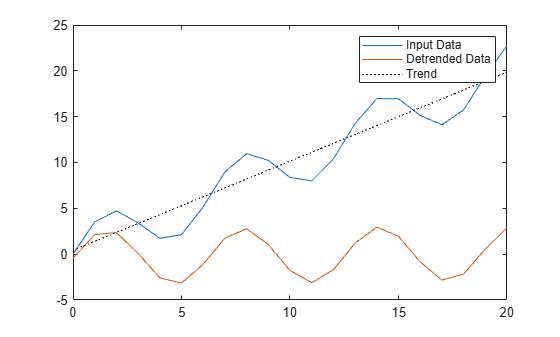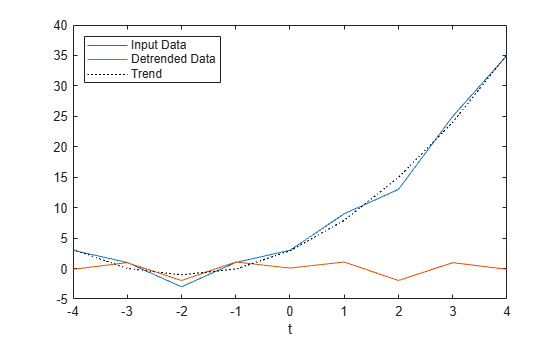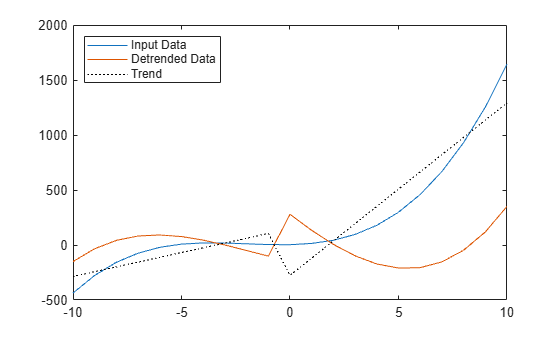# detrend

Remove polynomial trend

## Syntax

``D = detrend(A)``
``D = detrend(A,n)``
``D = detrend(A,n,bp)``
``D = detrend(___,nanflag)``
``D = detrend(___,Name,Value)``

## Description

example

````D = detrend(A)` removes the best straight-fit line from the data in `A` and returns the remaining data. If `A` is a vector, then `detrend` subtracts the trend from the elements of `A`.If `A` is a matrix, then `detrend` operates on each column separately, subtracting each trend from the corresponding column of `A`.If `A` is a multidimensional array, then `detrend` operates column-wise across all dimensions, subtracting each trend from the corresponding column of `A`.If `A` is a table or timetable with numeric variables of type `single` or `double`, then `detrend` operates on each variable of `A` separately, subtracting each trend from the corresponding variable of `A`. ```

example

````D = detrend(A,n)` removes the `n`th-degree polynomial trend. For example, when ```n = 0```, `detrend` removes the mean value from `A`. When `n = 1`, `detrend` removes the linear trend, which is equivalent to the previous syntax. When `n = 2`, `detrend` removes the quadratic trend.```

example

````D = detrend(A,n,bp)` removes a continuous, piecewise trend with segments defined by the breakpoints specified in the vector `bp`.```
````D = detrend(___,nanflag)` specifies how `NaN` values are treated for any of the previous syntaxes. For example, `detrend(A,"omitnan")` removes `NaN` values before calculating the trend, while `detrend(A,"includenan")` includes them (default).```

example

````D = detrend(___,Name,Value)` specifies additional parameters using one or more name-value arguments. For example, `detrend(A,1,bp,"Continuous",false)` specifies that the fitted trend can have discontinuities.```

## Examples

collapse all

Create a vector of data, and remove the continuous linear trend. Plot the original data, the detrended data, and the linear trend.

```t = 0:20; A = 3*sin(t) + t; D = detrend(A); plot(t,A) hold on plot(t,D) plot(t,A-D,":k") legend("Input Data","Detrended Data","Trend","Location","northwest")```Create a table of data, and remove the continuous quadratic trend from a specified variable in the table. Plot the original data, the detrended data, and the trend.

```t = (-4:4)'; trend = (t.^2 + 4*t + 3); sig = [0 1 -2 1 0 1 -2 1 0]'; x = sig + trend; T = table(t,trend,sig,x); T = detrend(T,2,"DataVariables","x","SamplePoints","t","ReplaceValues",false)```
```T=9×5 table t trend sig x x_detrended __ _____ ___ __ ___________ -4 3 0 3 -0.12121 -3 0 1 1 0.9697 -2 -1 -2 -3 -1.9654 -1 0 1 1 1.0736 0 3 0 3 0.08658 1 8 1 9 1.0736 2 15 -2 13 -1.9654 3 24 1 25 0.9697 4 35 0 35 -0.12121 ```
```plot(T,"t","x") hold on plot(T,"t","x_detrended") plot(T.t,T.x-T.x_detrended,":k") legend("Input Data","Detrended Data","Trend","Location","northwest")```Create a vector of data, and remove the piecewise linear trend using a breakpoint at 0. Specify that the resulting output can be discontinuous. Plot the original data, the detrended data, and the trend.

```t = -10:10; A = t.^3 + 6*t.^2 + 4*t + 3; bp = 0; D = detrend(A,1,bp,"SamplePoints",t,"Continuous",false); plot(t,A) hold on plot(t,D) plot(t,A-D,":k") legend("Input Data","Detrended Data","Trend","Location","northwest")```## Input Arguments

collapse all

Input data, specified as a vector, matrix, multidimensional array, table, or timetable.

• If `A` is a vector, then `detrend` subtracts the trend from the elements of `A`.

• If `A` is a matrix, then `detrend` operates on each column separately, subtracting each trend from the corresponding column of `A`.

• If `A` is a multidimensional array, then `detrend` operates column-wise across all dimensions, subtracting each trend from the corresponding column of `A`.

• If `A` is a table or timetable with numeric variables of type `single` or `double`, then `detrend` operates on each variable of `A` separately, subtracting each trend from the corresponding variable of `A`.

Data Types: `double` | `single`
Complex Number Support: Yes

Polynomial degree, specified as a nonnegative integer scalar, or as `"constant"` (equivalent to `0`) or `"linear"` (equivalent to `1`).

Breakpoints to define piecewise segments of the data, specified as a vector containing one of the following:

• Sample point values indicating the location of the breakpoints. Sample point values are contained either in the default sample points vector ```[1 2 3 ...]``` or specified by the `SamplePoints` name-value argument.

• Logical values where logical `1` (`true`) indicates a breakpoint in the corresponding element of the input data. If `bp` contains logical values, it must be the same length as the sample points.

Breakpoints are useful when you want to compute separate trends for different segments of the data.

Data Types: `double` | `single` | `datetime` | `duration` | `logical`

`NaN` condition, specified as one of these values:

• `"includenan"` — Include `NaN` values in the input data when computing the trend.

• `"omitnan"` — Ignore all `NaN` values in the input data when computing the trend.

### Name-Value Arguments

Specify optional pairs of arguments as `Name1=Value1,...,NameN=ValueN`, where `Name` is the argument name and `Value` is the corresponding value. Name-value arguments must appear after other arguments, but the order of the pairs does not matter.

Example: `D = detrend(A,SamplePoints=1:10:1000)`

Before R2021a, use commas to separate each name and value, and enclose `Name` in quotes.

Example: `D = detrend(A,"SamplePoints",1:10:1000)`

Continuity constraint, specified as one of these values:

• `true` — The fitted trend must be continuous everywhere.

• `false` — The fitted trend can contain discontinuities.

Sample points, specified as a vector of sample point values or one of the options in the following table when the input data is a table. The sample points represent the x-axis locations of the data, and must be sorted and contain unique elements. Sample points do not need to be uniformly sampled. The vector `[1 2 3 ...]` is the default.

When the input data is a table, you can specify the sample points as a table variable using one of these options:

Option for Table InputDescriptionExamples
Variable name

A character vector or scalar string specifying a single table variable name

`'Var1'`

`"Var1"`

Scalar variable index

A scalar table variable index

`3`

Logical vector

A logical vector whose elements each correspond to a table variable, where `true` specifies the corresponding variable as the sample points, and all other elements are `false`

`[true false false]`

Function handle

A function handle that takes a table variable as input and returns a logical scalar, which must be `true` for only one table variable

`@isnumeric`

`vartype` subscript

A table subscript generated by the `vartype` function that returns a subscript for only one variable

`vartype('numeric')`

Note

This name-value argument is not supported when the input data is a `timetable`. Timetables use the vector of row times as the sample points. To use different sample points, you must edit the timetable so that the row times contain the desired sample points.

Example: `detrend(A,"SamplePoints",0:0.1:10)`

Data Types: `double` | `single` | `datetime` | `duration`

Table or timetable variables to operate on, specified as one of the options in this table. The `DataVariables` value indicates for which variables of the input table or timetable to remove polynomial trends.

Other variables in the table or timetable not specified by `DataVariables` pass through to the output without being detrended.

OptionDescriptionExamples
Variable name

A character vector or string scalar specifying a single table variable name

`'Var1'`

`"Var1"`

Vector of variable names

A cell array of character vectors or string array, where each element is a table variable name

`{'Var1' 'Var2'}`

`["Var1" "Var2"]`

Scalar or vector of variable indices

A scalar or vector of table variable indices

`1`

`[1 3 5]`

Logical vector

A logical vector whose elements each correspond to a table variable, where `true` includes the corresponding variable and `false` excludes it

`[true false true]`

Function handle

A function handle that takes a table variable as input and returns a logical scalar

`@isnumeric`

`vartype` subscript

A table subscript generated by the `vartype` function

`vartype("numeric")`

For vector, matrix, or multidimensional array input data, `DataVariables` is not supported.

Example: ```detrend(A,"DataVariables",["Var1" "Var2" "Var4"])```

Replace values indicator, specified as one of these values when `A` is a table or timetable:

• `true` or `1` — Replace input table variables with table variables containing detrended data.

• `false` or `0` — Append input table variables with table variables containing detrended data.

For vector, matrix, or multidimensional array input data, `ReplaceValues` is not supported.

Example: `detrend(A,"ReplaceValues",false)`

## Tips

• The `detrend` function subtracts the mean or a best-fit line (in the least-squares sense) from your data. If your data is tabular or contains several data columns or is a table or timetable, `detrend` treats each data column separately.

Removing a trend from the data enables you to focus your analysis on the fluctuations in the data about the trend. A linear trend typically indicates a systematic increase or decrease in the data. A systematic shift can result from sensor drift, for example. While trends can be meaningful, some types of analyses yield better insight once you remove trends.

Whether it makes sense to remove trend effects in the data often depends on the objectives of your analysis.

## Version History

Introduced before R2006a

expand all